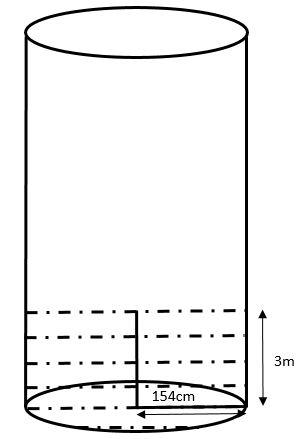QuestionAnswers

# A cylindrical tank has a radius of 154 cm. It is filled with water till a height of $3$ metres. If some water to a height of 4.5 metres is poured into it, what will be the increase in the volume of water?Hint: Here we have to calculate the increase in the volume of water, so we have to use the formula for volume of cylinder, $v=\pi {{r}^{2}}(H-h)$ where $h$, is the initial height of water filled in the jar and $H$ is the final height of water filled. We have the values of $h=3m$ and $H=4.5m$.

Complete step-by-step solution -
Here, we have a cylindrical tank with radius, $r=154cm$ which is filled with water till a height of 3 metres as in the figure:First we have to convert cm into m, we know that $1m=100cm$. Therefore, we can say that,
\begin{align} & 154cm=\dfrac{154}{100}m \\ & 154cm=1.54m \\ \end{align}
That is, we got $r=1.54m$
Initially, the height of the cylinder, $h=3m$.
After that we add water till it reaches a height, $H=4.5m$
So, here we have to calculate the increase in the volume of water.
We know that the formula for the volume of cylinder, $V$is:
$V=\pi {{r}^{2}}h$, where $r$ is the radius of the cylinder and $h$ is the height of the cylinder.
Here, for the height $h$, the volume of the cylinder denoted as ${{V}_{1}}$ is given by,
${{V}_{1}}=\pi {{r}^{2}}h$
Similarly, for the height $H$, the volume of the cylinder denoted by ${{V}_{2}}$ is given by,
${{V}_{2}}=\pi {{r}^{2}}H$
Hence, the increase in the volume of water, denoted by $v$ is given by,
\begin{align} & v={{V}_{2}}-{{V}_{1}} \\ & v=\pi {{r}^{2}}H-\pi {{r}^{2}}h \\ \end{align}
Now, by taking $\pi {{r}^{2}}$ outside we get:
$v=\pi {{r}^{2}}(H-h)$
In the next step, we have to substitute the values for $r=1.54,H=4$ and $h=3$. Thus, we get the equation:
\begin{align} & v=\pi \times {{(1.54)}^{2}}(4.5-3) \\ & v=\dfrac{22}{7}\times 1.54\times 1.54\times 1.5\text{ }....\text{ }\left( \pi =\dfrac{22}{7} \right) \\ \end{align}
By cancellation we obtain:
\begin{align} & v=22\times 0.22\times 1.54\times 1.5 \\ & v=4.84\times 1.54\times 1.5 \\ & v=7.4536\times 1.5 \\ & v=11.1804 \\ \end{align}
Hence, the increase in the volume of water is $11.1804{{m}^{3}}$

Note: Here, we have to calculate the increase in the volume of water, i.e. we have to subtract the volume of cylinder with height h from the volume of cylinder with height H. Since, it is increase in volume, don’t get confused that we have to add two volumes, it may lead to a wrong answer.

Where There is a Will There is a Way EssayArea of a Sector of a Circle FormulaVolume of a Triangular Prism FormulaThe Difference Between an Animal that is A Regulator and One that is A ConformerLaughter is a Best Medicine EssayElectric Field of a SphereArea of a Rhombus FormulaPerimeter of a Trapezoid FormulaSummary of a Photograph - PoemCentroid of a Trapezoid FormulaImportant Questions for CBSE Class 6 English A Pact with The Sun Chapter 10 - A Strange Wrestling MatchImportant Questions for CBSE Class 6 English A Pact with The Sun Chapter 1 - A Tale of Two BirdsImportant Questions for CBSE Class 6 English Honeysuckle Chapter 8 - A Game of ChanceImportant Questions for CBSE Class 11 Business Studies Chapter 7 - Formation of a CompanyImportant Questions for CBSE Class 7 English Honeycomb Chapter 2 - A Gift Of ChappalsImportant Questions for CBSE Class 6 English Honeysuckle Chapter 5 - A Different Kind of SchoolImportant Questions for CBSE Class 6 English A Pact with The Sun Chapter 8 - A Pact with the SunCBSE Class 7 Science Water: A Precious Resource WorksheetsImportant Questions for CBSE Class 11 Physics Chapter 4 - Motion in a PlaneImportant Questions for CBSE Class 6 English A Pact with The SunCBSE Class 10 Hindi A Question Paper 2020Hindi A Class 10 CBSE Question Paper 2009Hindi A Class 10 CBSE Question Paper 2015Hindi A Class 10 CBSE Question Paper 2016Hindi A Class 10 CBSE Question Paper 2012Hindi A Class 10 CBSE Question Paper 2010Hindi A Class 10 CBSE Question Paper 2008Hindi A Class 10 CBSE Question Paper 2014Hindi A Class 10 CBSE Question Paper 2007Hindi A Class 10 CBSE Question Paper 2013RS Aggarwal Class 8 Mathematics Solutions for Chapter-18 Area of a Trapezium and a PolygonNCERT Solutions for Class 9 English Moments Chapter 8 - A House is Not a HomeRD Sharma Solutions for Class 9 Maths Chapter 19 - Surface Area and Volume of A Right Circular CylinderNCERT Solutions for Class 12 Accountancy Chapter 4 - Reconstitution of a Partnership Firm:Retirement/Death of a PartnerNCERT Solutions for Class 12 Accountancy Chapter 3 - Reconstitution of a Partnership Firm: Admission of a PartnerNCERT Solutions for Class 10 English Footprints Without Feet Chapter 4 - A Question of TrustRD Sharma Solutions for Class 9 Maths Chapter 21 - Surface Area and Volume of a SphereRS Aggarwal Solutions Class 8 Chapter-18 Area of a Trapezium and a Polygon (Ex 18B) Exercise 18.2NCERT Solutions for Class 10 English Footprints Without Feet Chapter 1 - A Triumph of SurgeryRS Aggarwal Solutions Class 8 Chapter-18 Area of a Trapezium and a Polygon (Ex 18C) Exercise 18.3